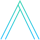# Eenie Meenie Miney Mo

July 10, 2019

Let’s write a function that let’s us take an n-sized subset of some iterator.

.css-1baulvz{display:inline-block;}function* take(n, iter) {  let index = 0    for (const x of iter) {    if (index >= n) return      index++    yield x  }}  const it = take(3, ["foo", "baz", "bar", "doe", "ray", "me"])printIterations(it) // foo, baz, bar

## .css-16kqvob{margin-left:-20px;padding-right:4px;color:#212121;}Cycling

Imagine you have an array, and you want to cycle through all the values, ad infinitum.

function* cycle(array) {  let index = 0    while (true) {    yield array[index]    index = (index + 1) % array.length  }}  const it = take(5, cycle([1, 2, 3]))printIterations(it) // 1, 2, 3, 1, 2

## Skipping

Now imagine you’re a teacher, and you are going to select a student for a task. However, each time you count, you skip a student.

// To skip 1 student means to add 2 with each iteration// To skip n students means to add n+1 with each iterationfunction* skip(n, array) {  /*   * A teacher would count the first student in the line as "1",   * and arrays are 0 indexed, so starting at -1 correct for that   *   * Notice that if you skip 0 students, you start at the beginning   * of the line, and count normally   */  let index = -1    while (true) {    index = (index + (n + 1)) % array.length    yield array[index]  }}  const it = take(  5,  skip(1, [    "Captain America",    "Black Widow",    "Hulk",    "Iron Man",    "Black Panther",  ]))printIterations(it)

// yes, your students are the Avengers.Black WidowIron ManCaptain AmericaHulkBlack Panther

How many times did we have to count until we got back to the start of the array?

function* skipUntilMatch(n, array, toFind) {  let index = -1  let count = 1    while (true) {    index = (index + (n + 1)) % array.length      if (array[index] === toFind) return count      yield    count++  }}  const arr = [  "Captain America",  "Black Widow",  "Hulk",  "Iron Man",  "Black Panther",]const it = skipUntilMatch(1, arr, arr)exhaustAndGrabLast(it) // 3, i.e. "miney"

## Generators

The functions were using above are called generators. They’re special functions that pause execution and yield a value. When they are executed the next() time, execution is resumed, as opposed to done over again, like a normal function. They are done when they finally return.

Generators are functions with the ability to pause and resume execution. A generator looks like a function but behaves like an iterator.

This gives us another way to iterate. One of the key functionalities provided is the ability to work with infinite series. When you iterate using a collection, all the values are sitting in memory, so you don’t have that ability.

function* naturalNumbers() {  let num = 1    while (true) {    yield num    num++  }}  const it = take(7, naturalNumbers())printIterations(it) // 1, 2, 3, 4, 5, 6, 7  /* or, if you don't want it to terminate */printIterations(naturalNumbers()) // 1, 2, 3, 4, ...

## Halving

Achilles is running a race. Each step is half the length of his previous step. How many steps until he’s not moving at all?

Theoretically, half of any number, ad infinitum, will never reach 0. Only it’s limit does.

However, in a programming language like JS, we’re dealing with finite resources, and that number will at some point reach 0. Let’s find out when.

function* halfUntilZero() {  let remaining = 1  let count = 0    while (true) {    if (remaining == 0) return count      yield    remaining = remaining / 2    count++  }}  exhaustAndGrabLast(halfUntilZero()) // 1075

Finally, let’s take a look at some of the helper functions we’ve been using. It might be useful to reflect on JavaScript’s iterator protocol.

function printIterations(it) {  let i = it.next()    while (!i.done) {    console.log(i.value)    i = it.next()  }}  function exhaustAndGrabLast(it) {  let current = it.next()  let value = current.value    while (!current.done) {    current = it.next()    value = current.value || value  }    return value}

## Sources

1. Understanding JavaScript Generators, inspired take and naturalNumbers
2. MDN